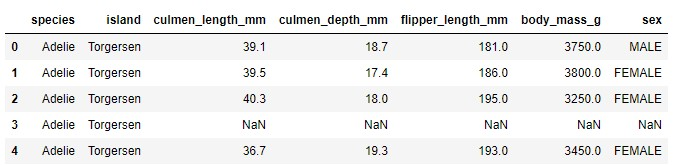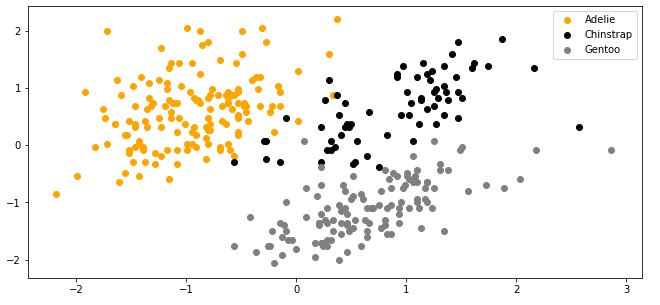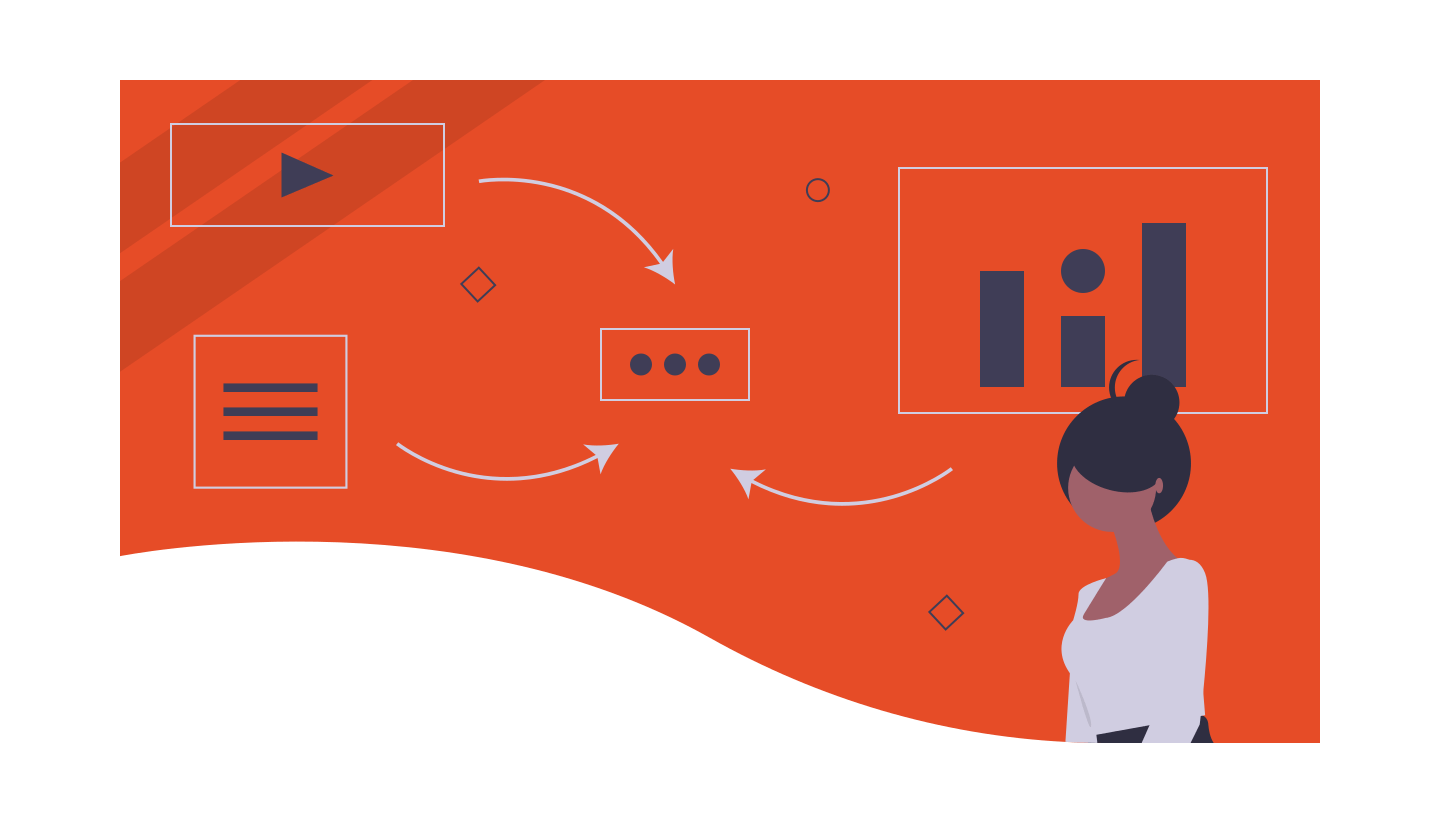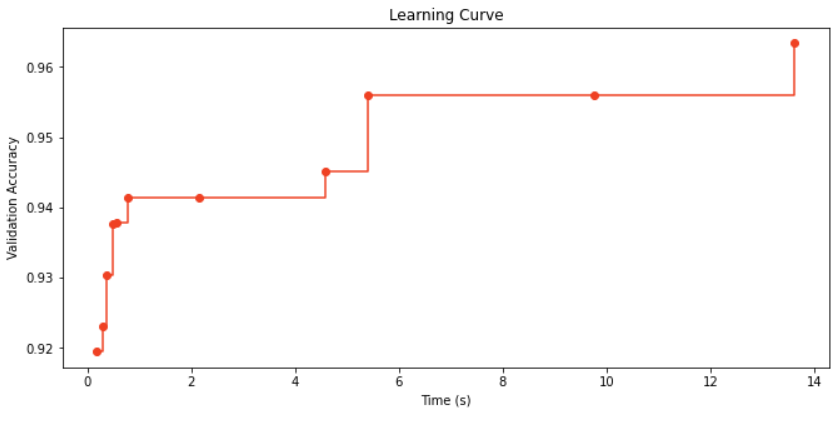Select Page

* indicates required

If you have ever worked on any Machine Learning project, you had a chance to witness how hard is to find a correct algorithm for your problem. An endless number of experiments with different hyperparameter optimization techniques need to be run in order to find the most suitable match.

This all increases the costs, computational resources and it is even worse for the environment. This gave rise to specific software solutions that are aiming to optimize this process – Automated Machine Learning (AutoML).

This bundle of e-books is specially crafted for beginners.
Everything from Python basics to the deployment of Machine Learning algorithms to production in one place.
Become a Machine Learning Superhero TODAY!

The goal of these solutions is to use low computational cost to search for algorithm and hyperparameter configurations that will and produce the optimal model. In a nutshell, AutoML tools are finding and training the best algorithm automatically. One of these tools is Python library FLAML.

1. Why choose FLAML for AutoML?
2. FLAML Installation
3. Data and Preparation
4. FLAML AutoML Training
5. FLAML AutoML Evaluation and Testing
6. Saving FLAML Model

## 1. Why choose FLAML for AutoML?

There are many AutoML libraries and tools out there, so let’s consider why should we use FLAML. Researchers from Microsoft built this library using several simple but effective techniques. Probably the most important one is their strategy for hyperparameter optimization – a blended search strategy. This strategy combines the strengths of global and local search and prioritizes them on the fly. This way the cost spent in finding good configurations is minimized. You can find more about this strategy in the paper here.The other cool thing about this library is the use of frugal optimization for cost-related hyperparameters. In essence, algorithms can have hyperparameters which can cause a large variation in the training cost.

Most of the hyperparameter optimization techniques don’t take this into consideration, meaning they are searching for the best results without taking costs as a viable benchmark. This technique is largely based on the randomized direct-search method, and you can learn more about it in the following paper.

FLAML library significantly outperforms top-ranked AutoML libraries on large open-source AutoML benchmarks. Also, this library is powering ML.NET Builder, an AutoML extension of ML.NET. If you want to dive deeper into FLAML you can do that by reading this paper.

## 2. FLAML Installation

In order to follow this tutorial, you need to have Python 3.6 or higher. Also, you need to install the FLAML library. That can be done using the following command:

``pip install flaml``

If you want to use FLAML within Jupyter Notebook like I do, additionally you need to run this command:

``pip install flaml[notebook]``In this tutorial, we utilize several libraries, so make sure you import them in the file:

``````import pandas as pd
import numpy as np
import matplotlib.pyplot as plt

from sklearn.model_selection import train_test_split
from sklearn.preprocessing import StandardScaler

from flaml import AutoML
from flaml.ml import sklearn_metric_loss_score
from flaml.data import get_output_from_log``````

## 3. Data and Preparation

Data that we use in this article is from PalmerPenguins Dataset. This dataset has been recently introduced as an alternative to the famous Iris dataset. It is created by Dr. Kristen Gorman and the Palmer Station, Antarctica LTER. You can obtain this dataset here, or via Kaggle.

This dataset is essentially composed of two datasets, each containing data of 344 penguins. Just like in Iris dataset there are 3 different species of penguins coming from 3 islands in the Palmer Archipelago. Also, these datasets contain culmen dimensions for each species. The culmen is the upper ridge of a bird’s bill. In the simplified penguin’s data, culmen length and depth are renamed as variables culmen_length_mm and culmen_depth_mm.Data itself is not too complicated. In essence, it is just tabular data:Note that in this tutorial, we ignore the species feature. This is because we perform unsupervised learning, ie. we don’t need the expected output value of the sample. We want our algorithm to figure that out on its own. Here is how data looks like when we plot it:## 4. FLAML AutoML Training

Ok, let’s start with the fun things. There are several libraries that we imported in the beginning and now it is time to put them to work. The most important class that we imported is AutoML from the FLAML library. This class is doing almost complete all job for us. Here is how we can use it for finding and training the most suitable algorithm for our problem:

``````automl = AutoML()

settings = {
"time_budget": 15,  # seconds
"metric": 'accuracy', # metric used for the evaluation
"log_file_name": "./penguins.log", # location of the log
}

automl.fit(X_train=X_train, y_train=y_train,
**settings)``````

First, we instantiate the object of AutoML class. Then we initialize the settings dictionary. This directory has several useful options we can use. The first option is time_budget and it defines how long the training will last.

The ‘metric’ option is used to define the metric which is going to be used for evaluating models during the training. The idea is to start training for multiple models with multiple hyperparameters configurations, evaluate them and pick the best.This option gives us the ability to define which metric is used for mentioned evaluation. This value is a string name or a function, and it can have several values: ‘accuracy’, ‘roc_auc’, ‘f1’, ‘micro_f1’, ‘macro_f1’, ‘log_loss’, ‘mae’, ‘mse’, ‘r2’. The “task” parameter defines the type of the task, eg., ‘classification’ or ‘regression’.

Finally, you can use log_file_name for defining the path where the training information will be stored. In our example, we use 15 seconds training period, accuracy for performance metric, and classification for a task.

After that, all you need to do is call the fit method on train and test data, and pass the settings dictionary. This will find the best algorithm and hyperparameters.

### 4.1 Training Output

You will notice that there is a lot of information that is printed out during this process. You can further investigate this output, but I will not go into details in this tutorial.

Apart from that, you may notice that a new file “penguins.log” appeared in the folder where the notebook is located. We use this file for evaluation later on. Here is the example of the log file:

``````{"record_id": 0, "iter_per_learner": 1, "logged_metric": false, "trial_time": 0.0974423885345459, "total_search_time": 0.18989896774291992, "validation_loss": 0.08053872053872055, "config": {"n_estimators": 4, "num_leaves": 4, "min_child_samples": 20, "learning_rate": 0.1, "subsample": 1.0, "log_max_bin": 8, "colsample_bytree": 1.0, "reg_alpha": 0.0009765625, "reg_lambda": 1.0}, "best_validation_loss": 0.08053872053872055, "best_config": {"n_estimators": 4, "num_leaves": 4, "min_child_samples": 20, "learning_rate": 0.1, "subsample": 1.0, "log_max_bin": 8, "colsample_bytree": 1.0, "reg_alpha": 0.0009765625, "reg_lambda": 1.0}, "learner": "lgbm", "sample_size": 273}
{"record_id": 1, "iter_per_learner": 3, "logged_metric": false, "trial_time": 0.040889739990234375, "total_search_time": 0.3075845241546631, "validation_loss": 0.07690235690235692, "config": {"n_estimators": 4, "num_leaves": 4, "min_child_samples": 12, "learning_rate": 0.2591253457286053, "subsample": 0.9266743941610592, "log_max_bin": 10, "colsample_bytree": 1.0, "reg_alpha": 0.0013933617380144255, "reg_lambda": 0.1809691794829294}, "best_validation_loss": 0.07690235690235692, "best_config": {"n_estimators": 4, "num_leaves": 4, "min_child_samples": 12, "learning_rate": 0.2591253457286053, "subsample": 0.9266743941610592, "log_max_bin": 10, "colsample_bytree": 1.0, "reg_alpha": 0.0013933617380144255, "reg_lambda": 0.1809691794829294}, "learner": "lgbm", "sample_size": 273}
{"record_id": 2, "iter_per_learner": 4, "logged_metric": false, "trial_time": 0.03989386558532715, "total_search_time": 0.36742496490478516, "validation_loss": 0.06956228956228958, "config": {"n_estimators": 4, "num_leaves": 4, "min_child_samples": 24, "learning_rate": 1.0, "subsample": 0.8513627344387319, "log_max_bin": 10, "colsample_bytree": 0.946138073111236, "reg_alpha": 0.0018311776973217071, "reg_lambda": 0.2790165919053837}, "best_validation_loss": 0.06956228956228958, "best_config": {"n_estimators": 4, "num_leaves": 4, "min_child_samples": 24, "learning_rate": 1.0, "subsample": 0.8513627344387319, "log_max_bin": 10, "colsample_bytree": 0.946138073111236, "reg_alpha": 0.0018311776973217071, "reg_lambda": 0.2790165919053837}, "learner": "lgbm", "sample_size": 273}
{"record_id": 3, "iter_per_learner": 6, "logged_metric": false, "trial_time": 0.04787254333496094, "total_search_time": 0.4881021976470947, "validation_loss": 0.062289562289562304, "config": {"n_estimators": 4, "num_leaves": 4, "min_child_samples": 16, "learning_rate": 0.5305016568114994, "subsample": 0.8132820472645405, "log_max_bin": 8, "colsample_bytree": 0.8110207792444197, "reg_alpha": 0.002464557255174736, "reg_lambda": 0.6369900700728733}, "best_validation_loss": 0.062289562289562304, "best_config": {"n_estimators": 4, "num_leaves": 4, "min_child_samples": 16, "learning_rate": 0.5305016568114994, "subsample": 0.8132820472645405, "log_max_bin": 8, "colsample_bytree": 0.8110207792444197, "reg_alpha": 0.002464557255174736, "reg_lambda": 0.6369900700728733}, "learner": "lgbm", "sample_size": 273}
{"record_id": 4, "iter_per_learner": 7, "logged_metric": false, "trial_time": 0.052857398986816406, "total_search_time": 0.5688858032226562, "validation_loss": 0.06222222222222222, "config": {"n_estimators": 7, "num_leaves": 4, "min_child_samples": 24, "learning_rate": 1.0, "subsample": 0.8513627344387319, "log_max_bin": 10, "colsample_bytree": 0.946138073111236, "reg_alpha": 0.0018311776973217073, "reg_lambda": 0.2790165919053837}, "best_validation_loss": 0.06222222222222222, "best_config": {"n_estimators": 7, "num_leaves": 4, "min_child_samples": 24, "learning_rate": 1.0, "subsample": 0.8513627344387319, "log_max_bin": 10, "colsample_bytree": 0.946138073111236, "reg_alpha": 0.0018311776973217073, "reg_lambda": 0.2790165919053837}, "learner": "lgbm", "sample_size": 273}
{"record_id": 5, "iter_per_learner": 9, "logged_metric": false, "trial_time": 0.10172533988952637, "total_search_time": 0.7853083610534668, "validation_loss": 0.058653198653198665, "config": {"n_estimators": 5, "num_leaves": 5, "min_child_samples": 19, "learning_rate": 0.38946718731417634, "subsample": 0.7593274397272737, "log_max_bin": 10, "colsample_bytree": 1.0, "reg_alpha": 0.0011281022212262847, "reg_lambda": 0.062447479285193244}, "best_validation_loss": 0.058653198653198665, "best_config": {"n_estimators": 5, "num_leaves": 5, "min_child_samples": 19, "learning_rate": 0.38946718731417634, "subsample": 0.7593274397272737, "log_max_bin": 10, "colsample_bytree": 1.0, "reg_alpha": 0.0011281022212262847, "reg_lambda": 0.062447479285193244}, "learner": "lgbm", "sample_size": 273}
{"record_id": 6, "iter_per_learner": 17, "logged_metric": false, "trial_time": 0.07380318641662598, "total_search_time": 2.150655746459961, "validation_loss": 0.05858585858585861, "config": {"n_estimators": 7, "num_leaves": 7, "min_child_samples": 10, "learning_rate": 0.6496763352968651, "subsample": 0.7765552597652422, "log_max_bin": 8, "colsample_bytree": 0.9158265282522311, "reg_alpha": 0.002034019632618961, "reg_lambda": 0.032548107388583214}, "best_validation_loss": 0.05858585858585861, "best_config": {"n_estimators": 7, "num_leaves": 7, "min_child_samples": 10, "learning_rate": 0.6496763352968651, "subsample": 0.7765552597652422, "log_max_bin": 8, "colsample_bytree": 0.9158265282522311, "reg_alpha": 0.002034019632618961, "reg_lambda": 0.032548107388583214}, "learner": "lgbm", "sample_size": 273}
``````

### 4.2 Training Results

Ok, let’s see which algorithm worked the best and what is the best hyperparameter configuration.

``````print('Best Machine Learning Algorithm:', automl.best_estimator)
print('Best hyperparmeter configuration:', automl.best_config)
print('Best accuracy on validation data: {0:.4g}'.format(1-automl.best_loss))
print('Training duration of best run: {0:.4g} s'.format(automl.best_config_train_time))``````
``````Best Machine Learning Algorithm: catboost
Best hyperparmeter configuration: {'early_stopping_rounds': 12, 'learning_rate': 0.1604199347807429}
Best accuracy on validation data: 0.956
Training duration of best run: 1.04 s``````

The output is a bit cryptic, but we can understand it. We can see that RandomForest was the best learner and that it uses 13 decision trees with 40 leaves with Gini impurity. This algorithm has an accuracy of 95% in the validation dataset. We can get most of this information by checking the property model.estimator as well:

``automl.model.estimator``
``````RandomForestClassifier(max_features=0.8499597562649218, max_leaf_nodes=40, n_estimators=13, n_jobs=-1)
``````

## 5. FLAML AutoML Evaluation and Testing

Next step would be evaluation on the test data. Here is how we can get predictions using FLAML:

``````y_pred = automl.predict(X_test)
print('Actual Values:', y_test)
print('Predictions:', y_pred)
``````
``````Actual Values: [0 1 0 0 2 0 1 0 1 0 0 0 1 0 0 1 2 1 1 1 0 0 0 1 1 1 1 1 0 1 1 1 0 2 1 2 2
0 1 1 1 1 0 0 1 1 0 1 2 2 1 0 0 2 1 0 2 1 0 0 2 0 0 1 1 0 1 0 1]
Predictions: [2 1 0 0 2 0 1 0 1 0 0 0 2 0 0 1 2 1 1 1 0 0 0 1 1 1 1 1 0 1 1 1 0 2 1 2 2
0 1 1 1 1 0 0 1 1 0 1 2 0 1 0 0 2 1 0 1 1 0 0 2 0 0 1 1 0 1 0 1]
``````

If we don’t want to calculate accuracy by hand, we can use sklearn_metric_loss_score method:

``````test_accuracy = 1 - sklearn_metric_loss_score('accuracy', y_pred, y_test)
print(f'Accuracy on test data: {test_accuracy}')
``````
``Accuracy on test data: 0.9710144927536232``

Another interesting thing that we can do is to explore log data that was stored during the training process.

### 5.1 Using FLAML Log Data for evaluation

Here is how we can utilize information that we have stored during the training time, by using method get_output_from_log:

``````plt.figure(figsize=(11, 5))
plt.title('Learning Curve')
plt.xlabel('Time (s)')
plt.ylabel('Validation Accuracy')
plt.scatter(time_history, 1 - np.array(valid_loss_history), c=RED)
plt.step(time_history, 1 - np.array(best_valid_loss_history), where='post', color=RED)
plt.show()``````## 6. Saving FLAML Model

In order to save FLAML Model, we can use Pickle Library. This Python library performs pickling on Python objects, ie. it implements binary protocols for serializing and de-serializing a Python object structure. Here is how we do that:

``````import pickle
with open('automl.pkl', 'wb') as f:
pickle.dump(automl, f, pickle.HIGHEST_PROTOCOL)``````

All done! Now you can find automl.plk file in your folder.

## Conclusion

In this article, we explored the fast and lightweight library FLAML. We applied it to Palmer Penguins Dataset and saw how we can use its many features. This library is quite useful and very quick, it will be a good addition to your Machine Learning toolbox.

This bundle of e-books is specially crafted for beginners.
Everything from Python basics to the deployment of Machine Learning algorithms to production in one place.
Become a Machine Learning Superhero TODAY!#### Nikola M. Zivkovic

Nikola M. Zivkovic is the author of books: Ultimate Guide to Machine Learning and Deep Learning for Programmers. He loves knowledge sharing, and he is an experienced speaker. You can find him speaking at meetups, conferences, and as a guest lecturer at the University of Novi Sad.

Ultimate Guide to Machine Learning with Python

Everything from Python basics to the deployment of Machine Learning algorithms to production in one place.

Become a Machine Learning Superhero TODAY!